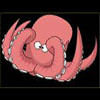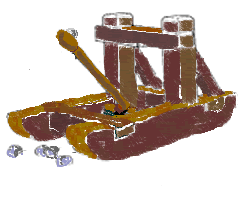#### You may also like### Base Puzzle

This investigation is about happy numbers in the World of the Octopus where all numbers are written in base 8 .Octi the octopus counts.### Happy Octopus

This investigation is about happy numbers in the World of the Octopus where all numbers are written in base 8 ... Find all the fixed points and cycles for the happy number sequences in base 8.### Basically

The number 3723(in base 10) is written as 123 in another base. What is that base?

# Oh for the Mathematics of Yesteryear

##### Age 11 to 14Challenge LevelA garrison of $600$ men has just enough bread to allow $24$ ounces a day to each man for $35$ days; but, with the news that the enemy was planning an attack, the garrison was forced to reinforce to $4 800$ men. The enemy arrives and decides to enforce a siege - to reduce their own loss of life. How many ounces of bread a day must each man in the garrison be allowed, to hold out $45$ days against the siege of the enemy?

If the transport of $60$ cwt. (hundredweights) of bread for $20$ miles costs the garrison $£14 \; 10\text{s}$. (i.e. Fourteen pounds ten shillings), what weight can be carried $30$ miles for $£5 \; 8\text{s } 9 \text{d}$?

n.b. in those days:

$12$d(pennies) = $1$s (shilling)

$20$s = $£1$ (pound)

$16$ ounces = $1$ lb (pound - "weight")

$112$ lb= $1$ cwt